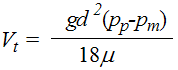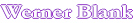Home                               Sedimentation of Spherical Particles in Liquid This program uses the Stokes equation to calculate the sedimentation (settling) speed of spherical particles The Stokes equation permits the calculation of the drag forces of a spherical particle in a viscous liquid at very low Reynolds numbers. Stoke's equation is derived from the generally not solvable Navier-Stokes equation.Vt is the settling or terminal speed of the particle, g is the acceleration of the spherical particle, d is the diameter of the particle, pp is the density of the particle, pm the density of the liquid medium, µ is the dynamic viscosity of the medium. The diameter of the particle is the Stoke's diameter, which is not the actual diameter of the particle, but rather the diameter effected by absorption, hydration and deviation from the spherical shape. For non-spherical particle the Stoke's diameter can be smaller than the actual diameter. Absorption of polymers on a particle will increase the Stoke's diameter. Input Select  Units Enter data Conversion Units Acceleration of gravity (g) cm/s2 ft/s2 inch/s2 m/s2 m/s2 Particle diameter (d) cm m micron nanometer m Density of particle (pp) g/cm3 g/l g/m3 kg/m3 lb/ft3 g/m3 Density of medium (pm) g/cm3 g/l g/m3 kg/m3 lb/ft3 g/m3 Viscosity of medium (µ) cP (cps) g.m-1.s-1 kg.m-1.s-1 mPa.s Pa.s Poise g.m-1.s-1 Results Settling Speed (Vt ) cm/sec m/sec micron/sec nanometer/sec Acheson, D. J. (1990), Elementary Fluid Dynamics, Oxford Applied Mathematics and Computing Science Series, Oxford University Press, ISBN 0198596790

Automatic recalculation

 Last edited on: October 20, 2010 Copyright®, Design, Layout and Technical Content by: